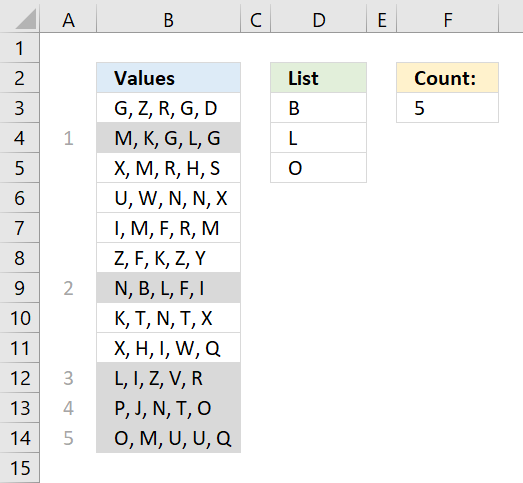Author: Oscar Cronquist Article last updated on October 09, 2018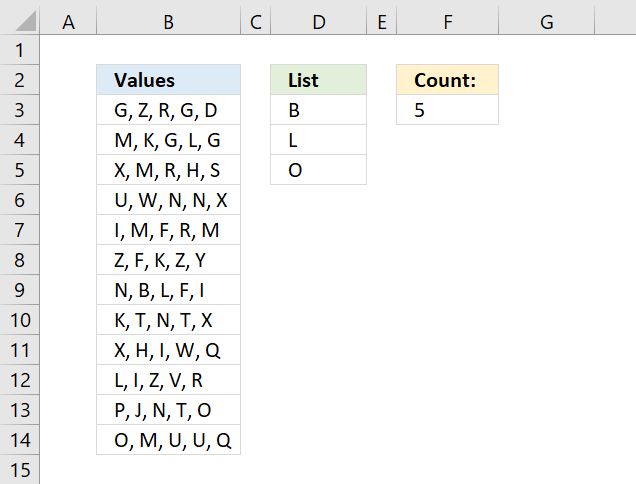The array formula in cell F3 counts cells in column B that contains at least one of the values in D3:D5. Each cell is only counted once.

=SUM((MMULT(ISNUMBER(SEARCH(TRANSPOSE(D3:D5), B3:B14))*1, ROW(D3:D5)^0)>0)*1)

To enter the array formula, type the formula in a cell then press and hold CTRL + SHIFT simultaneously, now press Enter once. Release all keys.

The formula bar now shows the formula with a beginning and ending curly bracket telling you that you entered the formula successfully. Don't enter the curly brackets yourself.

### Explaining the formula

The TRANSPOSE function changes the values in D3:D5 from being vertically arranged to being horizontally arranged.

TRANSPOSE(D3:D5)

Note the semicolon and comma characters that separate the values below.

{"B";"L";"O"} => {"B","L","O"}

The SEARCH function requires the values to be arranged in one column in the first argument and in one row in the second argument or vice versa.

That is why the TRANSPOSE function is needed, you could, of course, enter the values horizontally on the worksheet to avoid the TRANSPOSE function.

SEARCH(TRANSPOSE(D3:D5), B3:B14) returns the following array, displayed in the picture below.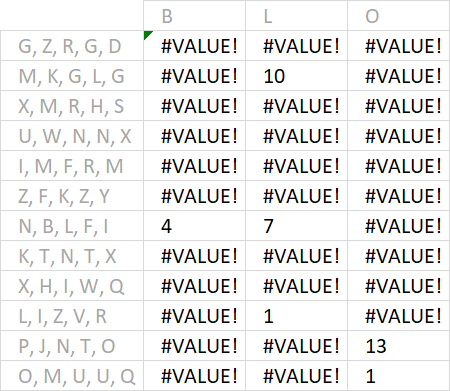Example, B is found in character position 4 in text string N, B, L, F, I. Note that the SEARCH function returns a #VALUE error if nothing is found.

The ISNUMBER function returns TRUE or FALSE determined by a value is a number or not, it happily ignores errors.

ISNUMBER(SEARCH(TRANSPOSE(D3:D5), B3:B14)) returns the following array.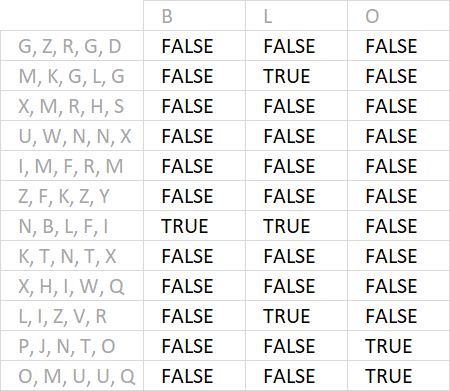The MMULT function sums the values row by row and returns an array shown in the picture below.

MMULT(ISNUMBER(SEARCH(TRANSPOSE(D3:D5), B3:B14))*1, ROW(D3:D5)^0)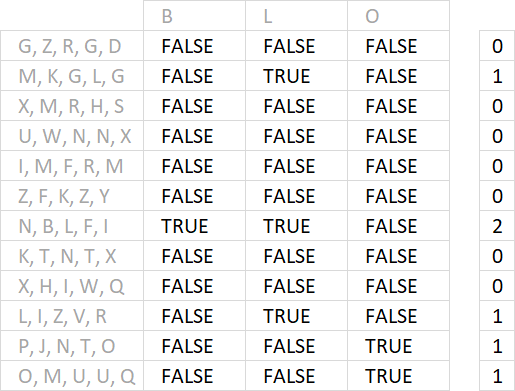To be able to do that we must use this array as the second argument: {1;1;1} It is determined by the number of cells in the list, in this case, three. They must be 1 and arranged vertically.

That is why I built this formula that builds the array automatically: ROW(D3:D5)^0

The MMULT function can't work with boolean values so I multiply them all by 1 to convert them into 0 (zeros) or 1.

The next thing is to check if the values in the array are larger than 0 (zero).

MMULT(ISNUMBER(SEARCH(TRANSPOSE(D3:D5), B3:B14))*1, ROW(D3:D5)^0)>0

Lastly, the SUM function adds the numbers and returns a total in cell F3.